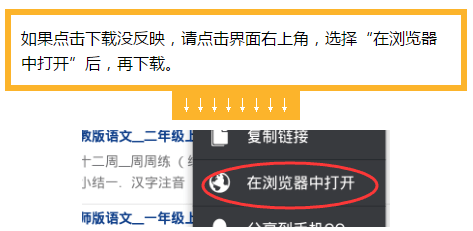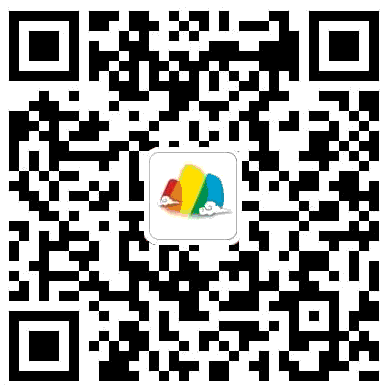﻿ 北师版数学__一年级上__第十二周__周周练 - 数学 - 智慧山

# 北师版数学__一年级上__第十二周__周周练

2015-11-23 13:24:00

4103

1

1.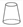这是一个圆柱。      （    ）

2. 黑板面一定是正方体。 （    ）

3.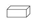这是一个长方体。（    ）

4. 苹果是球。                   （    ）

2正方体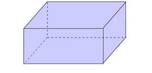长方体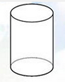球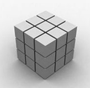圆柱

3

3+1=          4+2=          7-2=

6+3=          10-1=         9-8=

1+5=          9+0=          1+7=

2+6=         10-5=          9-3=

3+4=         7-0=            8-3=

4

1. 长方体、正方体都有（  ）个面。

4,6

2. 正方体的每个面都是（     ）。

（正方形，长方形）

3. 球上（   ）平平的面。

（有，没有）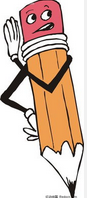9     9     1

6     9     8

8     5     6

7     7     5

2. 正方形

3. 没有[点击下载周周练]